# 1.6 Absolute value functions  (Page 5/7)

 Page 5 / 7

Solve $\text{\hspace{0.17em}}-2|k-4|\le -6.$

$k\le 1\text{\hspace{0.17em}}$ or $\text{\hspace{0.17em}}k\ge 7;\text{\hspace{0.17em}}$ in interval notation, this would be $\text{\hspace{0.17em}}\left(-\infty ,1\right]\cup \left[7,\infty \right)$

## Key concepts

• The absolute value function is commonly used to measure distances between points. See [link] .
• Applied problems, such as ranges of possible values, can also be solved using the absolute value function. See [link] .
• The graph of the absolute value function resembles a letter V. It has a corner point at which the graph changes direction. See [link] .
• In an absolute value equation, an unknown variable is the input of an absolute value function.
• If the absolute value of an expression is set equal to a positive number, expect two solutions for the unknown variable. See [link] .
• An absolute value equation may have one solution, two solutions, or no solutions. See [link] .
• An absolute value inequality is similar to an absolute value equation but takes the form It can be solved by determining the boundaries of the solution set and then testing which segments are in the set. See [link] .
• Absolute value inequalities can also be solved graphically. See [link] .

## Verbal

How do you solve an absolute value equation?

Isolate the absolute value term so that the equation is of the form $\text{\hspace{0.17em}}|A|=B.\text{\hspace{0.17em}}$ Form one equation by setting the expression inside the absolute value symbol, $\text{\hspace{0.17em}}A,\text{\hspace{0.17em}}$ equal to the expression on the other side of the equation, $\text{\hspace{0.17em}}B.\text{\hspace{0.17em}}$ Form a second equation by setting $\text{\hspace{0.17em}}A\text{\hspace{0.17em}}$ equal to the opposite of the expression on the other side of the equation, $\text{\hspace{0.17em}}-B.\text{\hspace{0.17em}}$ Solve each equation for the variable.

How can you tell whether an absolute value function has two x -intercepts without graphing the function?

When solving an absolute value function, the isolated absolute value term is equal to a negative number. What does that tell you about the graph of the absolute value function?

The graph of the absolute value function does not cross the $\text{\hspace{0.17em}}x$ -axis, so the graph is either completely above or completely below the $\text{\hspace{0.17em}}x$ -axis.

How can you use the graph of an absolute value function to determine the x -values for which the function values are negative?

How do you solve an absolute value inequality algebraically?

First determine the boundary points by finding the solution(s) of the equation. Use the boundary points to form possible solution intervals. Choose a test value in each interval to determine which values satisfy the inequality.

## Algebraic

Describe all numbers $\text{\hspace{0.17em}}x\text{\hspace{0.17em}}$ that are at a distance of 4 from the number 8. Express this using absolute value notation.

Describe all numbers $\text{\hspace{0.17em}}x\text{\hspace{0.17em}}$ that are at a distance of $\text{\hspace{0.17em}}\frac{1}{2}\text{\hspace{0.17em}}$ from the number −4. Express this using absolute value notation.

$\text{\hspace{0.17em}}|x+4|=\frac{1}{2}\text{\hspace{0.17em}}$

Describe the situation in which the distance that point $\text{\hspace{0.17em}}x\text{\hspace{0.17em}}$ is from 10 is at least 15 units. Express this using absolute value notation.

Find all function values $\text{\hspace{0.17em}}f\left(x\right)\text{\hspace{0.17em}}$ such that the distance from $\text{\hspace{0.17em}}f\left(x\right)\text{\hspace{0.17em}}$ to the value 8 is less than 0.03 units. Express this using absolute value notation.

$|f\left(x\right)-8|<0.03$

For the following exercises, solve the equations below and express the answer using set notation.

$|x+3|=9$

$|6-x|=5$

$\left\{1,11\right\}$

$|5x-2|=11$

$|4x-2|=11$

$\left\{\frac{9}{4},\text{\hspace{0.17em}}\text{\hspace{0.17em}}\text{\hspace{0.17em}}\text{\hspace{0.17em}}\text{\hspace{0.17em}}\frac{13}{4}\right\}$

$2|4-x|=7$

$3|5-x|=5$

$\left\{\frac{10}{3},\text{\hspace{0.17em}}\text{\hspace{0.17em}}\text{\hspace{0.17em}}\text{\hspace{0.17em}}\text{\hspace{0.17em}}\frac{20}{3}\right\}$

$3|x+1|-4=5$

$5|x-4|-7=2$

$\left\{\frac{11}{5},\text{\hspace{0.17em}}\text{\hspace{0.17em}}\text{\hspace{0.17em}}\text{\hspace{0.17em}}\text{\hspace{0.17em}}\frac{29}{5}\right\}$

$0=-|x-3|+2$

$2|x-3|+1=2$

$\left\{\frac{5}{2},\frac{7}{2}\right\}$

$|3x-2|=7$

$|3x-2|=-7$

No solution

$|\frac{1}{2}x-5|=11$

$|\frac{1}{3}x+5|=14$

$\left\{-57,27\right\}$

$-|\frac{1}{3}x+5|+14=0$

For the following exercises, find the x - and y -intercepts of the graphs of each function.

$f\left(x\right)=2|x+1|-10$

$\left(0,-8\right);\text{\hspace{0.17em}}\left(-6,0\right),\text{\hspace{0.17em}}\left(4,0\right)$

$f\left(x\right)=4|x-3|+4$

$f\left(x\right)=-3|x-2|-1$

$\left(0,-7\right);\text{\hspace{0.17em}}$ no $\text{\hspace{0.17em}}x$ -intercepts

$f\left(x\right)=-2|x+1|+6$

For the following exercises, solve each inequality and write the solution in interval notation.

$|x-2|>10$

$\left(-\infty ,-8\right)\cup \left(12,\infty \right)$

$2|v-7|-4\ge 42$

$|3x-4|\le 8$

$\frac{-4}{3}\le x\le 4$

$|x-4|\ge 8$

$|3x-5|\ge 13$

$\left(-\infty ,-\frac{8}{3}\right]\cup \left[6,\infty \right)$

$|3x-5|\ge -13$

$|\frac{3}{4}x-5|\ge 7$

$\left(-\infty ,-\frac{8}{3}\right]\cup \left[16,\infty \right)$

$|\frac{3}{4}x-5|+1\le 16$

## Graphical

For the following exercises, graph the absolute value function. Plot at least five points by hand for each graph.

$y=|x-1|$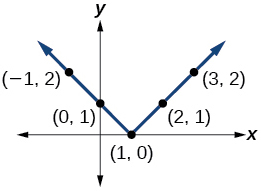$y=|x+1|$

$y=|x|+1$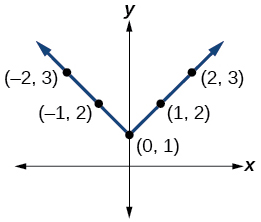For the following exercises, graph the given functions by hand.

$y=|x|-2$

$y=-|x|$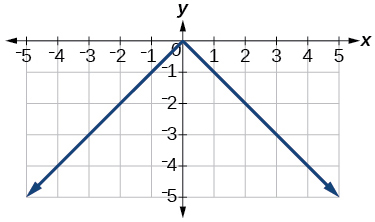$y=-|x|-2$

$y=-|x-3|-2$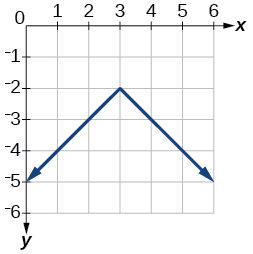$f\left(x\right)=-|x-1|-2$

$f\left(x\right)=-|x+3|+4$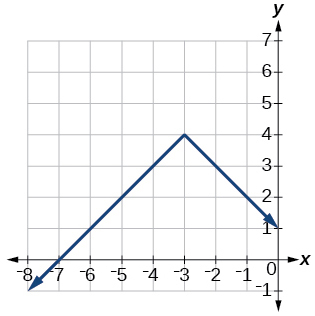$f\left(x\right)=2|x+3|+1$

$f\left(x\right)=3|x-2|+3$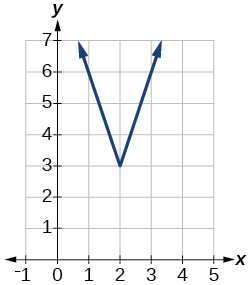$f\left(x\right)=|2x-4|-3$

$f\left(x\right)=|3x+9|+2$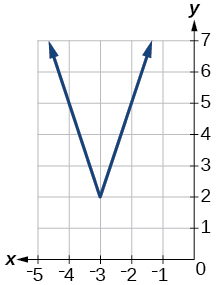$f\left(x\right)=-|x-1|-3$

$f\left(x\right)=-|x+4|-3$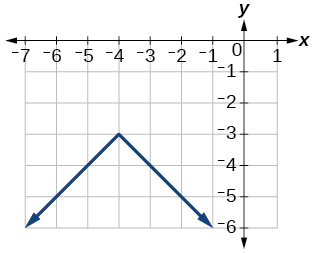$f\left(x\right)=\frac{1}{2}|x+4|-3$

## Technology

Use a graphing utility to graph $f\left(x\right)=10|x-2|$ on the viewing window $\left[0,4\right].$ Identify the corresponding range. Show the graph.

range: $\text{\hspace{0.17em}}\left[0,20\right]$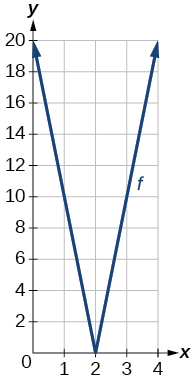Use a graphing utility to graph $\text{\hspace{0.17em}}f\left(x\right)=-100|x|+100\text{\hspace{0.17em}}$ on the viewing window $\text{\hspace{0.17em}}\left[-5,5\right].\text{\hspace{0.17em}}$ Identify the corresponding range. Show the graph.

For the following exercises, graph each function using a graphing utility. Specify the viewing window.

$f\left(x\right)=-0.1|0.1\left(0.2-x\right)|+0.3$

$x\text{-}$ intercepts: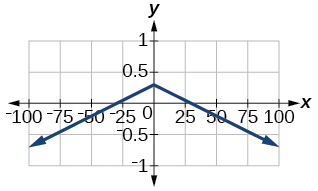$f\left(x\right)=4×{10}^{9}|x-\left(5×{10}^{9}\right)|+2×{10}^{9}$

## Extensions

For the following exercises, solve the inequality.

$|-2x-\frac{2}{3}\left(x+1\right)|+3>-1$

$\left(-\infty ,\infty \right)$

If possible, find all values of $a$ such that there are no $x\text{-}$ intercepts for $f\left(x\right)=2|x+1|+a.$

If possible, find all values of $\text{\hspace{0.17em}}a\text{\hspace{0.17em}}$ such that there are no $\text{\hspace{0.17em}}y$ -intercepts for $\text{\hspace{0.17em}}f\left(x\right)=2|x+1|+a.$

There is no solution for $\text{\hspace{0.17em}}a\text{\hspace{0.17em}}$ that will keep the function from having a $\text{\hspace{0.17em}}y$ -intercept. The absolute value function always crosses the $\text{\hspace{0.17em}}y$ -intercept when $\text{\hspace{0.17em}}x=0.$

## Real-world applications

Cities A and B are on the same east-west line. Assume that city A is located at the origin. If the distance from city A to city B is at least 100 miles and $\text{\hspace{0.17em}}x\text{\hspace{0.17em}}$ represents the distance from city B to city A, express this using absolute value notation.

The true proportion $\text{\hspace{0.17em}}p\text{\hspace{0.17em}}$ of people who give a favorable rating to Congress is 8% with a margin of error of 1.5%. Describe this statement using an absolute value equation.

$|p-0.08|\le 0.015$

Students who score within 18 points of the number 82 will pass a particular test. Write this statement using absolute value notation and use the variable $\text{\hspace{0.17em}}x\text{\hspace{0.17em}}$ for the score.

A machinist must produce a bearing that is within 0.01 inches of the correct diameter of 5.0 inches. Using $\text{\hspace{0.17em}}x\text{\hspace{0.17em}}$ as the diameter of the bearing, write this statement using absolute value notation.

$|x-5.0|\le 0.01$

The tolerance for a ball bearing is 0.01. If the true diameter of the bearing is to be 2.0 inches and the measured value of the diameter is $\text{\hspace{0.17em}}x\text{\hspace{0.17em}}$ inches, express the tolerance using absolute value notation.

can you not take the square root of a negative number
No because a negative times a negative is a positive. No matter what you do you can never multiply the same number by itself and end with a negative
lurverkitten
Actually you can. you get what's called an Imaginary number denoted by i which is represented on the complex plane. The reply above would be correct if we were still confined to the "real" number line.
Liam
Suppose P= {-3,1,3} Q={-3,-2-1} and R= {-2,2,3}.what is the intersection
can I get some pretty basic questions
In what way does set notation relate to function notation
Ama
is precalculus needed to take caculus
It depends on what you already know. Just test yourself with some precalculus questions. If you find them easy, you're good to go.
Spiro
the solution doesn't seem right for this problem
what is the domain of f(x)=x-4/x^2-2x-15 then
x is different from -5&3
Seid
All real x except 5 and - 3
Spiro
***youtu.be/ESxOXfh2Poc
Loree
how to prroved cos⁴x-sin⁴x= cos²x-sin²x are equal
Don't think that you can.
Elliott
By using some imaginary no.
Tanmay
how do you provided cos⁴x-sin⁴x = cos²x-sin²x are equal
What are the question marks for?
Elliott
Someone should please solve it for me Add 2over ×+3 +y-4 over 5 simplify (×+a)with square root of two -×root 2 all over a multiply 1over ×-y{(×-y)(×+y)} over ×y
For the first question, I got (3y-2)/15 Second one, I got Root 2 Third one, I got 1/(y to the fourth power) I dont if it's right cause I can barely understand the question.
Is under distribute property, inverse function, algebra and addition and multiplication function; so is a combined question
Abena
find the equation of the line if m=3, and b=-2
graph the following linear equation using intercepts method. 2x+y=4
Ashley
how
Wargod
what?
John
ok, one moment
UriEl
how do I post your graph for you?
UriEl
it won't let me send an image?
UriEl
also for the first one... y=mx+b so.... y=3x-2
UriEl
y=mx+b you were already given the 'm' and 'b'. so.. y=3x-2
Tommy
Please were did you get y=mx+b from
Abena
y=mx+b is the formula of a straight line. where m = the slope & b = where the line crosses the y-axis. In this case, being that the "m" and "b", are given, all you have to do is plug them into the formula to complete the equation.
Tommy
thanks Tommy
Nimo
0=3x-2 2=3x x=3/2 then . y=3/2X-2 I think
Given
co ordinates for x x=0,(-2,0) x=1,(1,1) x=2,(2,4)
neil
"7"has an open circle and "10"has a filled in circle who can I have a set builder notation
Where do the rays point?
Spiro
x=-b+_Гb2-(4ac) ______________ 2a
I've run into this: x = r*cos(angle1 + angle2) Which expands to: x = r(cos(angle1)*cos(angle2) - sin(angle1)*sin(angle2)) The r value confuses me here, because distributing it makes: (r*cos(angle2))(cos(angle1) - (r*sin(angle2))(sin(angle1)) How does this make sense? Why does the r distribute once
so good
abdikarin
this is an identity when 2 adding two angles within a cosine. it's called the cosine sum formula. there is also a different formula when cosine has an angle minus another angle it's called the sum and difference formulas and they are under any list of trig identities
strategies to form the general term
carlmark
consider r(a+b) = ra + rb. The a and b are the trig identity.
Mike
How can you tell what type of parent function a graph is ?
generally by how the graph looks and understanding what the base parent functions look like and perform on a graph
William
if you have a graphed line, you can have an idea by how the directions of the line turns, i.e. negative, positive, zero
William
y=x will obviously be a straight line with a zero slope
William
y=x^2 will have a parabolic line opening to positive infinity on both sides of the y axis vice versa with y=-x^2 you'll have both ends of the parabolic line pointing downward heading to negative infinity on both sides of the y axis
William
y=x will be a straight line, but it will have a slope of one. Remember, if y=1 then x=1, so for every unit you rise you move over positively one unit. To get a straight line with a slope of 0, set y=1 or any integer.
Aaron
yes, correction on my end, I meant slope of 1 instead of slope of 0
William
what is f(x)=
I don't understand
Joe
Typically a function 'f' will take 'x' as input, and produce 'y' as output. As 'f(x)=y'. According to Google, "The range of a function is the complete set of all possible resulting values of the dependent variable (y, usually), after we have substituted the domain."
Thomas
Sorry, I don't know where the "Â"s came from. They shouldn't be there. Just ignore them. :-)
Thomas
Darius
Thanks.
Thomas
Â
Thomas
It is the Â that should not be there. It doesn't seem to show if encloses in quotation marks. "Â" or 'Â' ... Â
Thomas
Now it shows, go figure?
ThomasByByBy Sean WiffleBoyBy Stephen VoronBy Alec MoffitBy Vanessa SoledadBy Briana HamiltonBy Briana KnowltonBy Nicole DuquetteBy RhodesBy DanielrosenbergerBy Janet Forrester